login  home  contents  what's new  discussion  bug reports help  links  subscribe  changes  refresh  edit

 Submitted by : (unknown) at: 2007-11-17T22:22:01-08:00 (15 years ago) Name : Axiom Version : default friCAS-20090114 Axiom-20050901 OpenAxiom-20091012 OpenAxiom-20110220 OpenAxiom-Release-141 Category : Axiom Aldor Interface Axiom Compiler Axiom Library Axiom Interpreter Axiom Documentation Axiom User Interface building Axiom from source lisp system MathAction Doyen CD Reduce Axiom on Windows Axiom on Linux Severity : critical serious normal minor wishlist Status : open closed rejected not reproducible fix proposed fixed somewhere duplicate need more info Optional subject :   Optional comment :

When I type:

fricas
(1) -> ex1:=(x-x/(x+exp(x)/(x*log(x+x))))*exp(2)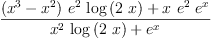(1)
Type: Expression(Integer)
fricas
ex2:=D(ex1, x)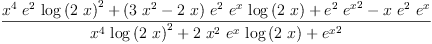(2)
Type: Expression(Integer)
fricas
ex3:=simplify(ex2)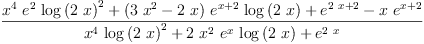(3)
Type: Expression(Integer)
fricas
integrate(ex3, x)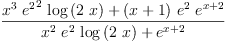(4)
Type: Union(Expression(Integer),...)

then Axiom 3.9 returns unevaluated integral.

Note, that if I remove the exp(2) factor, then Axiom is able to compute the integral.

fricas
ex4:=(x-x/(x+exp(x)/(x*log(x+x))))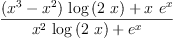(5)
Type: Expression(Integer)
fricas
ex5:=D(ex4, x)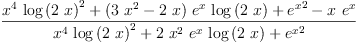(6)
Type: Expression(Integer)
fricas
ex6:=simplify(ex5)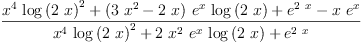(7)
Type: Expression(Integer)
fricas
integrate(ex6, x)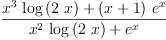(8)
Type: Union(Expression(Integer),...)

We claim that Axiom is good at integration, therefore critical --kratt6, Wed, 10 May 2006 01:28:56 -0500 reply
Severity: normal => critical

We claim that Axiom is good at integration, therefore critical --kratt6, Wed, 10 May 2006 01:29:14 -0500 reply
Category: Axiom Mathematics => Axiom Library

Related example --unknown, Wed, 10 May 2006 06:22:25 -0500 reply
If I try:
fricas
integrate(simplify(((x^4*log(2*x)^2+(3*x^2-2*x)*exp(x)*log(2*x)+exp(2*x)-x*exp(x))/(x^4*log(2*x)^2+2*x^2*exp(x)*log(2*x)+exp(2*x))-1)*exp(2)), x)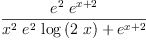(9)
Type: Union(Expression(Integer),...)

I see that Axiom failed to recognize that e^4 - (e^2)^2 is zero. AFAICS similar problem appears in original example: when I trace rischNormalize I see that both e^2 and e^4 appear in "normalized" function. But this may cause trouble later, since Axiom assumes that different kernels are algebraically independent. I do not know if rischNormalize is supposed to simplify constants (maybe this shoud be done by a separtate pass) but failing to recognize "transcendental" zero may give wrong results and otherwise Risch algorithm may declare an integrable function as non integrable.

fixed in FriCAS revision 475 --kratt6, Fri, 10 Apr 2009 03:20:47 -0700 reply
Status: open => fixed somewhere

Status: fixed somewhere => closed

 Subject:   Be Bold !! ( 14 subscribers )